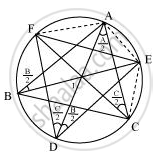Share

# Bisectors of angles A, B and C of a triangle ABC intersect its circumcircle at D, E and F respectively. Prove that the angles of the triangle DEF are - CBSE Class 9 - Mathematics

#### Question

Bisectors of angles A, B and C of a triangle ABC intersect its circumcircle at D, E and F respectively. Prove that the angles of the triangle DEF are 90^@-1/2A, 90^@-1/2B" and "90^@-1/2C

#### SolutionIt is given that BE is the bisector of ∠B.

∴ ∠ABE = ∠B/2

However, ∠ADE = ∠ABE (Angles in the same segment for chord AE)

Similarly, ∠ACF = ∠ADF = ∠C/2         (Angle in the same segment for chord AF)

=(angleB)/2 + (angleC)/2

=1/2(angleB+angleC)

=1/2(180^@-angleA)

=90^@-1/2angleA

Similarly, it can be proved that

angleE=90^@-1/2angleB

angleF=90^@-1/2angleC

Is there an error in this question or solution?

#### APPEARS IN

NCERT Solution for Mathematics Class 9 (2018 to Current)
Chapter 10: Circles
Ex. 10.60 | Q: 8 | Page no. 187

#### Video TutorialsVIEW ALL 

Solution Bisectors of angles A, B and C of a triangle ABC intersect its circumcircle at D, E and F respectively. Prove that the angles of the triangle DEF are Concept: Cyclic Quadrilaterals.
S